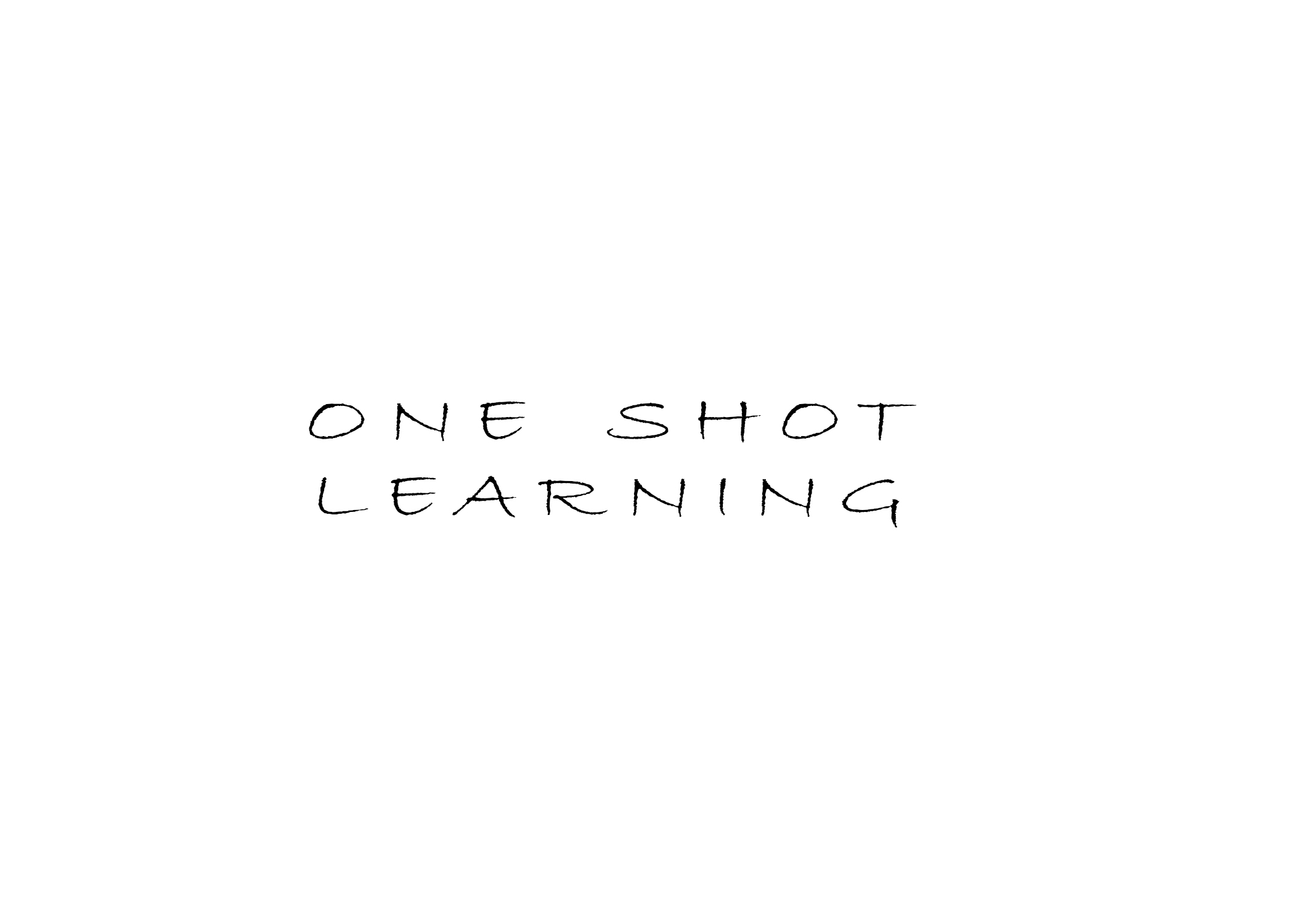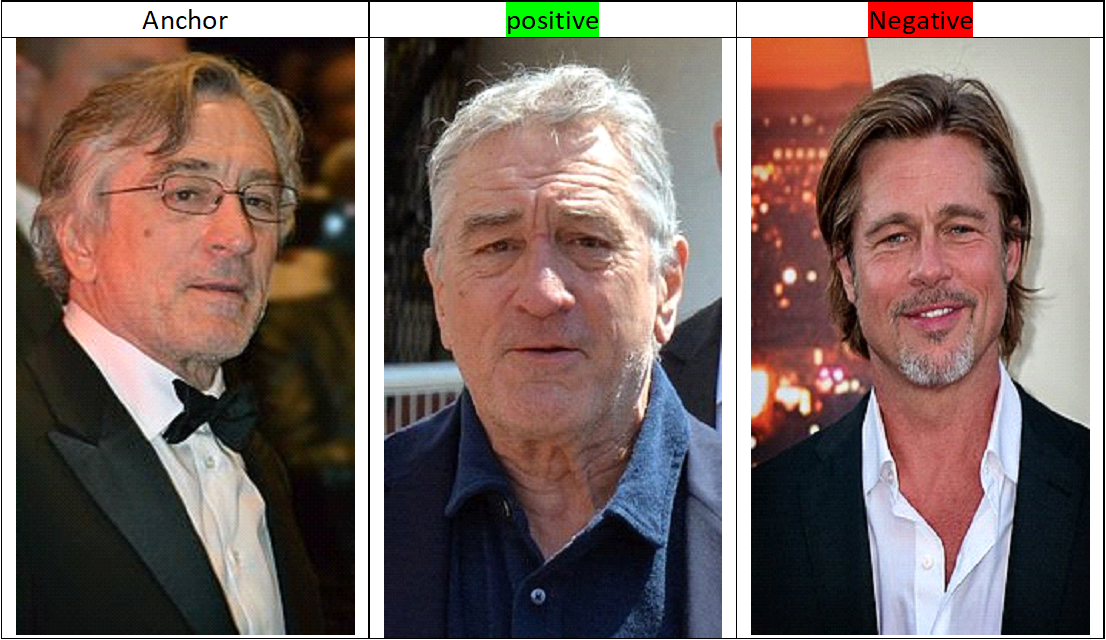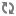## One-Shot Learning

One of the challenges of face recognition is solving the "One shot learning" problem – recognizing a person using one example of his face. As we all know "conventional" supervised learning doesn't work on 1 training example per class, therefore we need to find a different way of tackling this problem in order to solve it. In this article we would learn how to solve this problem using the paper "Siamese Neural Networks for One-shot Image Recognition" (Gregory et al., 2015).

First, we need to define the problems we want to solve – face recognition and face verification. Face verification is checking whether the person checked is the claimed person?  For example, putting your passport in a scanning machine that verifies that you are the "correct person". Face Recognition is checking "who is this person?". We would see how this problem can be solved via solving the verification problem.

In order to solve the face verification problem in a one-shot fashion, we'd have to learn a "difference function", i.e. difference (img1, img2) = degree of difference between images. If the difference (img1, img2) is lower than a threshold than we would say that the images are similar otherwise they are different. So how can we measure the difference? Answer – Norm of the encodings' difference.

The practical way of defining encoding is to represent the picture by a vector (you might be familiar with one hot encoding for labels). In order to represent the picture in a meaningful fashion we'd use the output of a fully connected layer from a Convolutional neural network, usually the last one. You can pick a CNN of your liking (VGG16 etc.).

Training the neural network requires a different approach; because on one side we want to penalize if the encoding of the checked picture (Anchor) is different from the encoding of the correct picture (Positive) encoding; but on the other side we want the penalize if the encoding of the Anchor is similar to the encoding of a wrong picture (Negative) encoding. We would use the same encoding for all the pictures i.e. using the same CNN on all pictures.From the requirements above we get the following loss function:

(1) J=∑i=1m[||f(A(i)) -f(P(i))||-||f(A(i)) -f(N(i))|| ]

Where: J – loss function, m – batch size,  f() – the fully connected layer output,

A(i) – i-th anchor picture,

P(i)  – i-th positive picture ,

N(i) –  i-th Negative picture

We have 2 problems with the definition of this loss function. The first one is that the f can be a function that converts the picture to a vector of zeros. We can solve it by adding a constant (margin) to the Loss function thus eliminating the trivial solution of zeros. The second one is that the loss function needs to be bigger or equal to zero. Therefore, we get the following loss function where α is the margin:

J=∑max(i=1m[||f(A(i)) -f(P(i))||-||f(A(i)) -f(N(i))|| +α],0)

Code in Python using TensorFlow 1.14 as tf:

 def triplet_loss(anchor_encoding, positive_encoding, negative_encoding, alpha):    """ Triplet loss as defined by formula (2)    Returns:  value of the loss function"""    # Step 1: Computing the distance between the anchor and the positive    pos_dist = tf.reduce_sum(tf.square(tf.subtract(anchor_encoding, positive_encoding)),axis =-1)    # Step 2: Computing the distance between the anchor and the negative    neg_dist = tf.reduce_sum(tf.square(tf.subtract(anchor_encoding, negative_encoding)),axis =-1)    # Step 3: Subtracting the distances and adding alpha.    temp_loss = tf.add(tf.subtract(pos_dist, neg_dist), alpha)    # Step 4: Check maximum between temp_loss and 0.0. and summing over training examples.    loss = tf.reduce_sum(tf.maximum(0.0, temp_loss))    return loss

With the trained model, we can pick a threshold for the verification of the person like 0.7 and see the result of the difference function after running the picture through the neural network. Solving the face recognition problem requires us to check the similarity of the anchor with all the pictures we have in the database. The decision clause would be whichever anchor has the lowest difference is the one picked to be the label. We can also choose a threshold, so that if neural network isn't sure that the person exists then it'll label it as "unknown person".

After reading all this you can guess why this paper's Neural network is called "Siamese Neural Network". It's because we must compare the anchor twice (once with positive and once with negative) in order to calculate the loss function.

There are a lot of ways to look at a problem and defining the loss function. The better you define it the better the results you'll get.

Hope you've learned a lot,

Don Shaked

הגיע הזמן לתפקיד הבא בקריירה? יש לנו עשרות משרות אטרקטיביות בתחום ה Data scientist תוכל/י לצפות במשרות כאן
או להשאיר פרטים (דיסקרטיות מלאה מובטחת!!)